﻿ 一种限速切断阀的结构设计及试验研究
 舰船科学技术2018, Vol. 40Issue (3): 76-82PDF

Structure design and experimental research on a type of speed limiting shut-off valve
ZHANG Tao, GUI Wen-bin, YANG Yi, LIU Jin-rong
Shanghai Marine Equipment Research Institute, Shanghai 200031, China
Abstract: According to the needs of marine engineering equipment, designed a special speed limiting shut-off valve. First of all, the flow path design and theoretical calculation of main structural parameters of the speed limiting shut-off valve is carried out, and the UG software is used to simulate the components of the speed limiting shut-off valve. Secondly, a new experimental method is proposed which is to use the small diameter cylinder drive the large diameter cylinder to produce the large flow to close the speed limiting shut-off valve, and the front and rear damping hole diameter's influence on the speed limiting shut-off valve and the system performance is carried out. The test results show that the speed limiting shut-off valve meet the needs of marine engineering equipment in terms of shut-off time and shut-off flow rate, and the diameter of the front and rear orifice has a significant impact on the dynamic performance of the speed limiting shut-off valve. The new experiment plan can provide reference for the same type of valve experiment, the conclusion of the experiment can provide guidance for the development of the same type of speed limiting shut-off valve.
Key words: shut-off valve     structure design     dynamic performance     experimental research
0 引　言

1 限速切断阀的结构设计 1.1 流道结构设计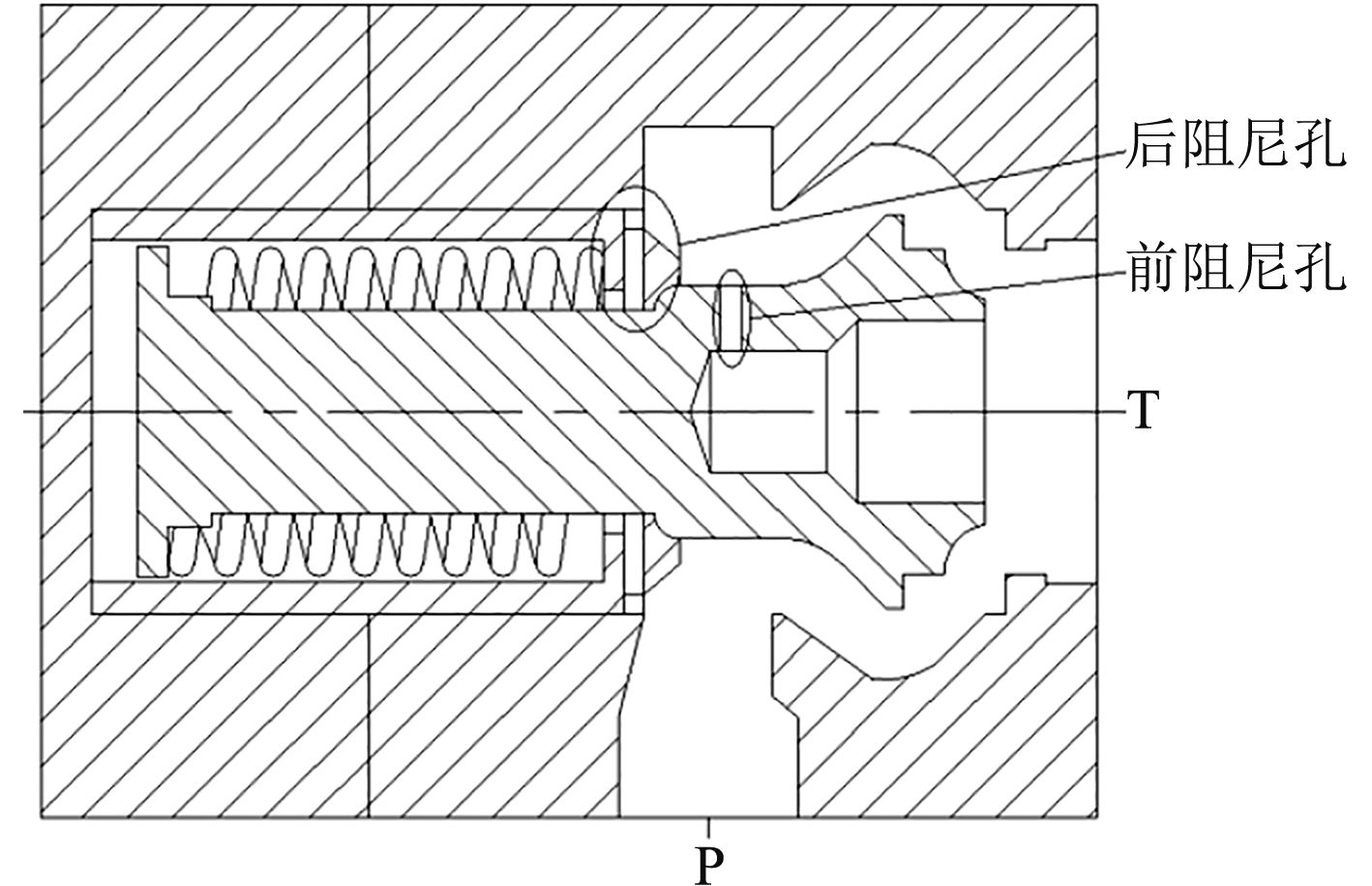图 1 限速切断阀机械结构简图 Fig. 1 Mechanical structure diagram of speed limiting shut-off valve

1.2 主要参数设计计算

1）进出油口直径的计算

 $d \geqslant 0.463\sqrt {\frac{Q}{V}} \text{，}$ (1)

 $d \geqslant 0.463\sqrt {\frac{Q}{V}} = 0.463\sqrt {\frac{{2200}}{8}} = 76.8 \ {{{mm}}} \text{，}$

 $d = 77 \ {{{mm}}} \text{。}$

2）渐缩型流道起始处的过流面积计算

 ${A_c} = \frac{Q}{V} = \frac{{2\;200}}{{60 \times 10}} \times {10^{ - 3}} = 3\;666.7 \ {{m}}{{{m}}^2} \text{，}$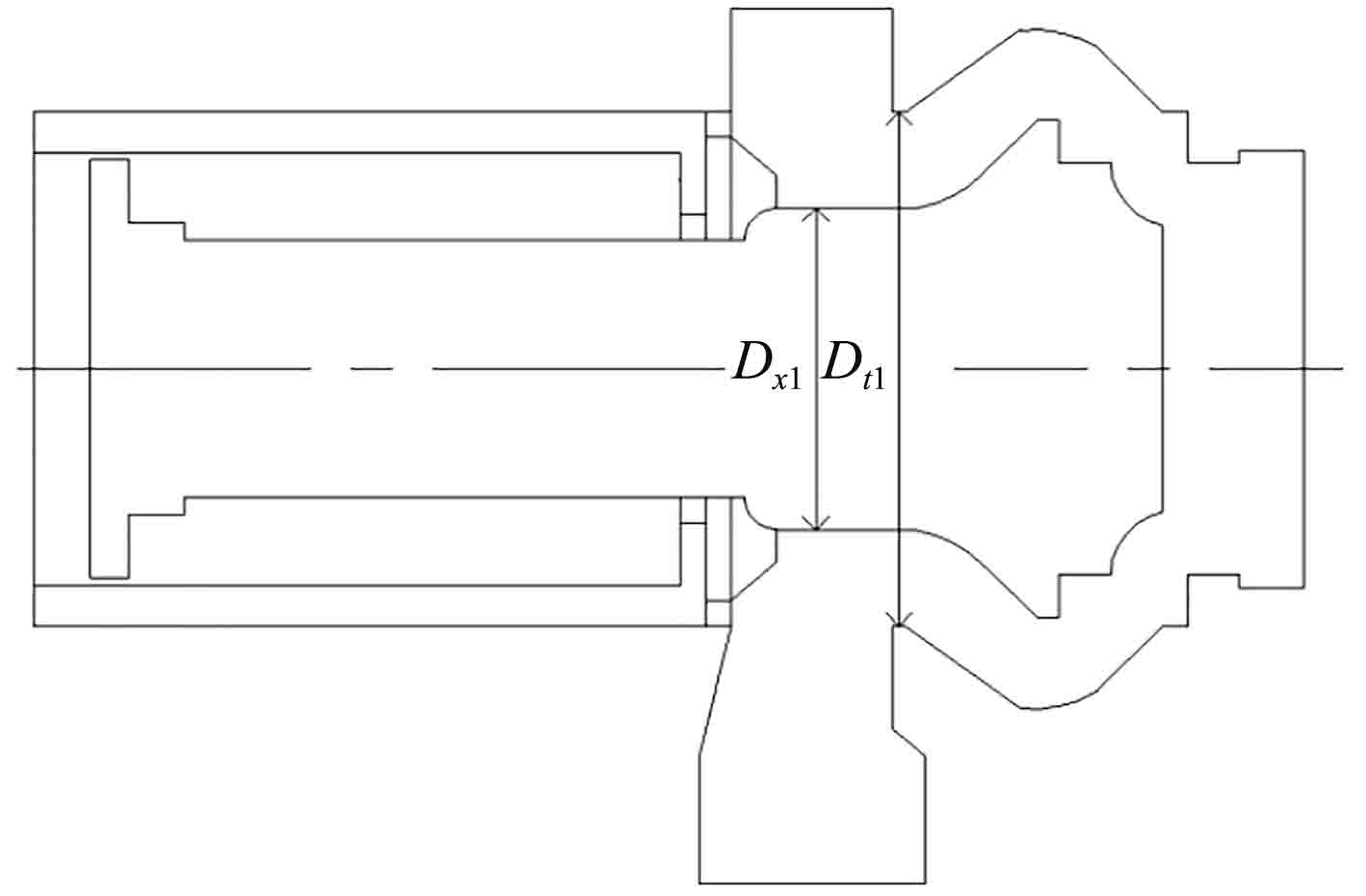图 2 渐缩型流道起始处尺寸示意图 Fig. 2 Schematic diagram of the beginning size of the tapered flow path

 ${A_c} = \frac{{{\pi }}}{4}\left( {D_{t1}^2 - D_{x1}^2} \right) = 3\;694.5 \ {{{m}}{{{m}}^2}} \text{。}$

3）阀芯直径的设计计算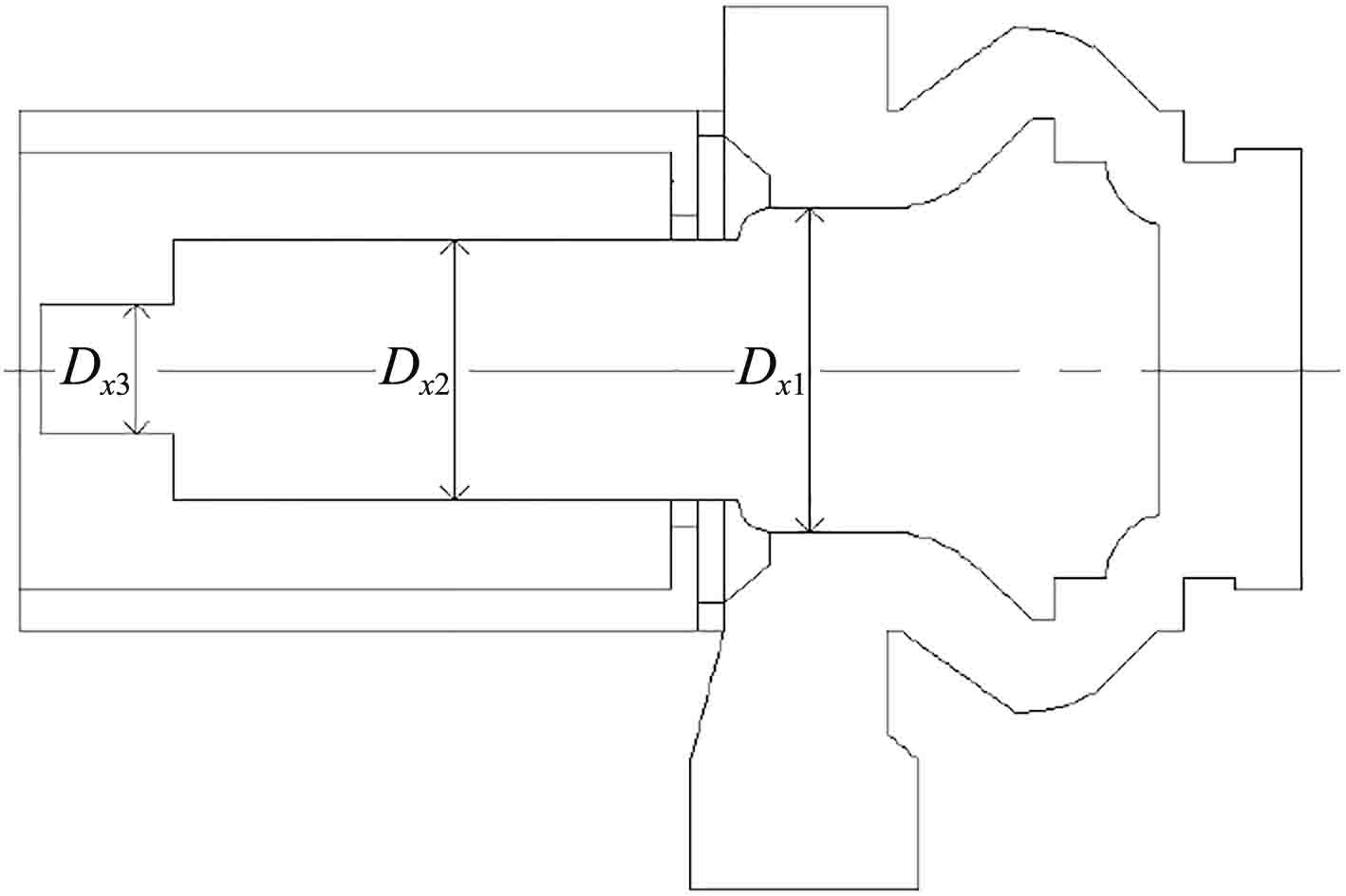图 3 阀芯各段尺寸示意图 Fig. 3 Schematic diagram of all spool section size

4）前阻尼孔直径计算

 ${Q_z} = {C_d}{A_z}\sqrt {\frac{2}{\rho }\Delta {P_z}} \text{，}$ (2)

 ${d_z} = \sqrt {\frac{{{Q_z}}}{{{C_d} \cdot \displaystyle\frac{\pi }{4} \cdot \sqrt {\frac{2}{\rho }\Delta {P_z}} }}} = 4{{mm}} \text{。}$

5）弹簧预压力及弹簧刚度的计算

 ${F_s} = {F_k} + {F_w} \text{。}$ (3)

 ${F_k} = \Delta {P_k} \times {A_k} \text{，}$ (4)

 ${F_w} = {P_w} \times {A_w} \text{，}$ (5)

 ${F_k} = \Delta {P_k} \times {A_k} = 599.85 {{N}} \text{。}$

 ${F_w} = {P_w} \times {A_w} = 146.08 {{N}} \text{。}$

 ${F_s} = {F_k} + {F_w} = 745.93 {{N}} \text{。}$

 ${F_s} = {K_s}{X_0} \text{。}$ (6)

 ${X_0} = 1 \sim 5{X_m} \text{。}$ (7)

 ${K_s} = 15 {{{N}}/{{mm}}} \text{。}$

6）主流道部分的设计计算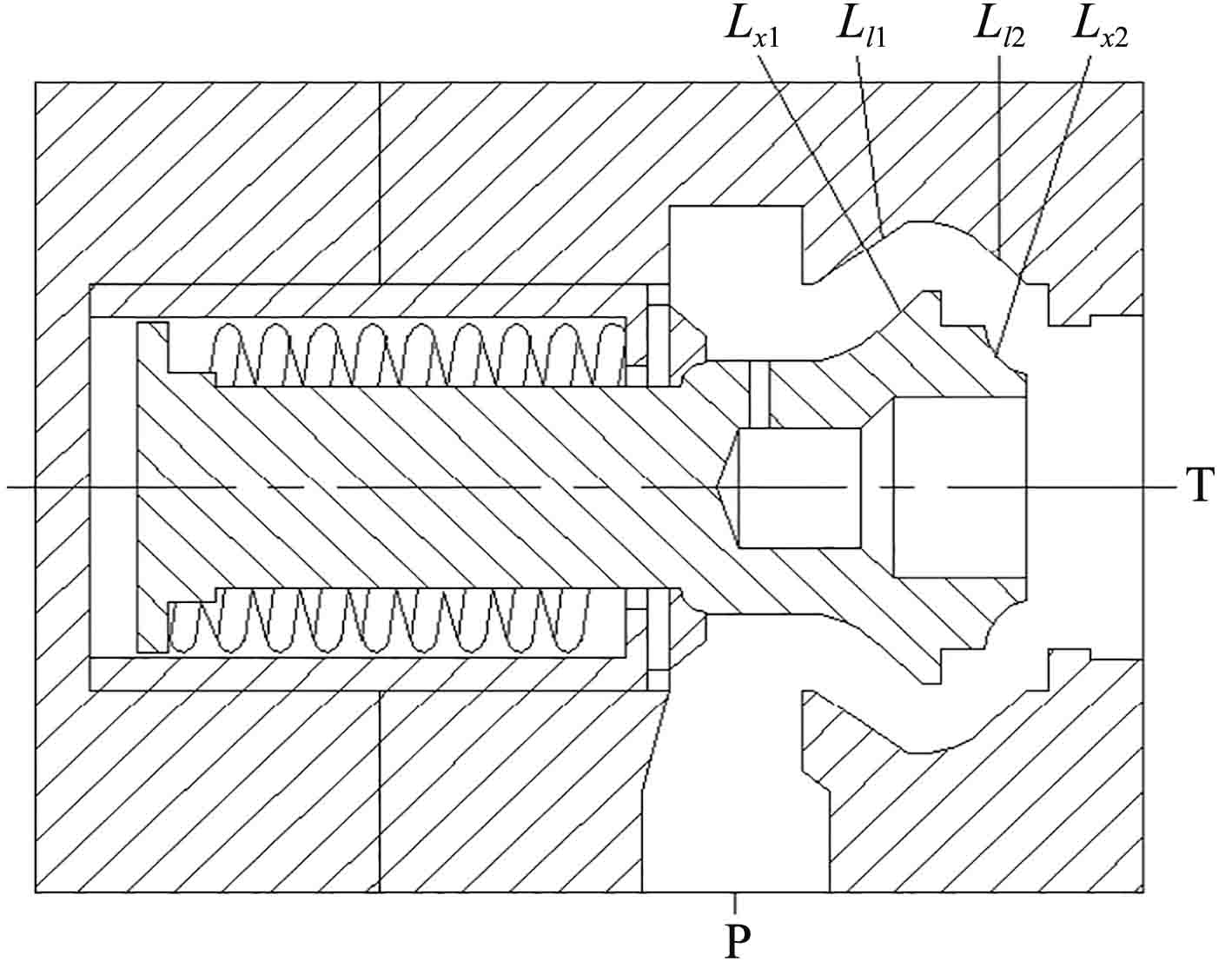图 4 标明研究曲线的限速切断阀示意图 Fig. 4 Schematic diagram of Speed limiting shut-off valve with indicating study curves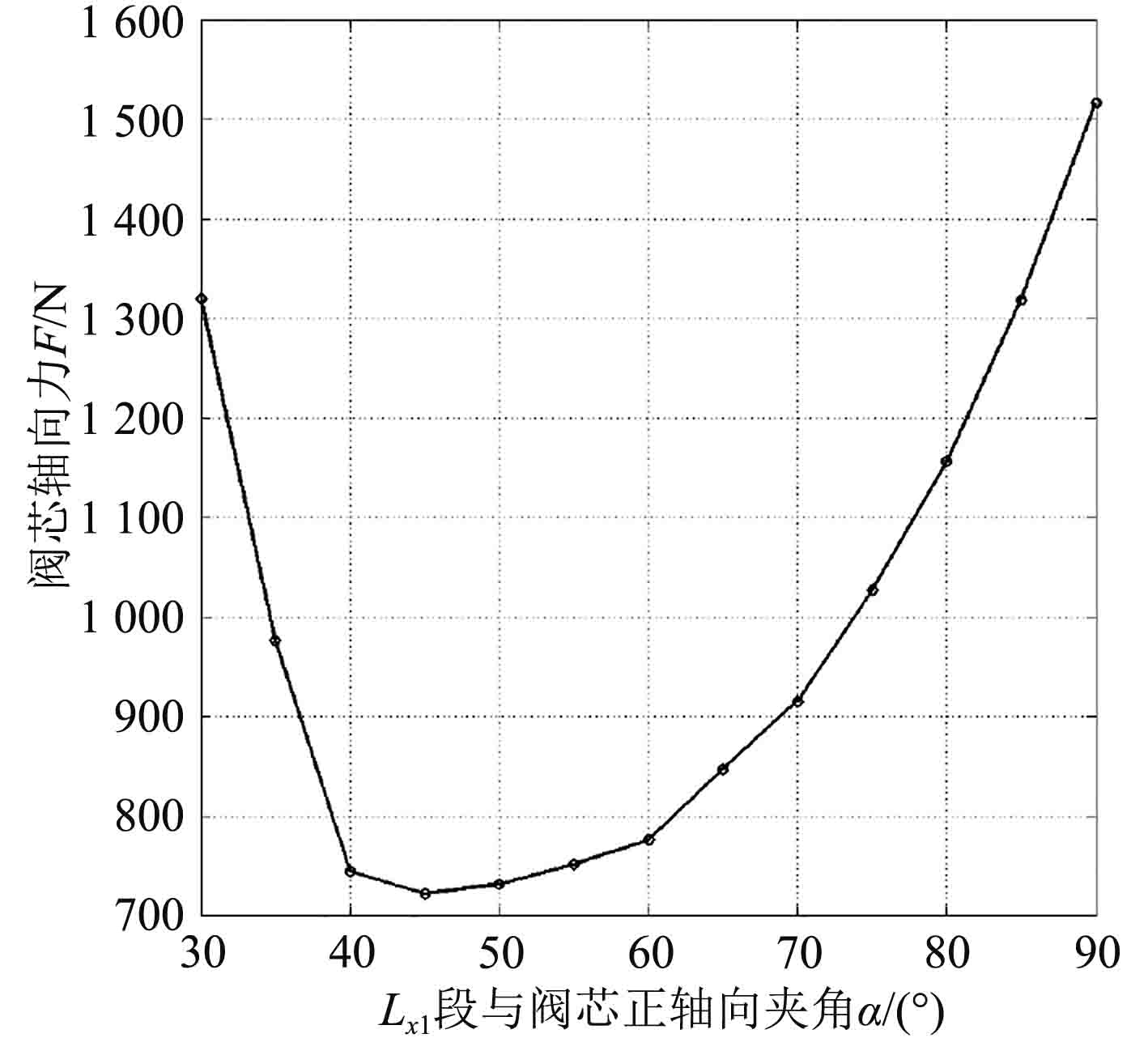图 5 阀芯轴向液压力与夹角α的关系曲线图 Fig. 5 The relationship curve of valve axial hydraulic pressure and angle α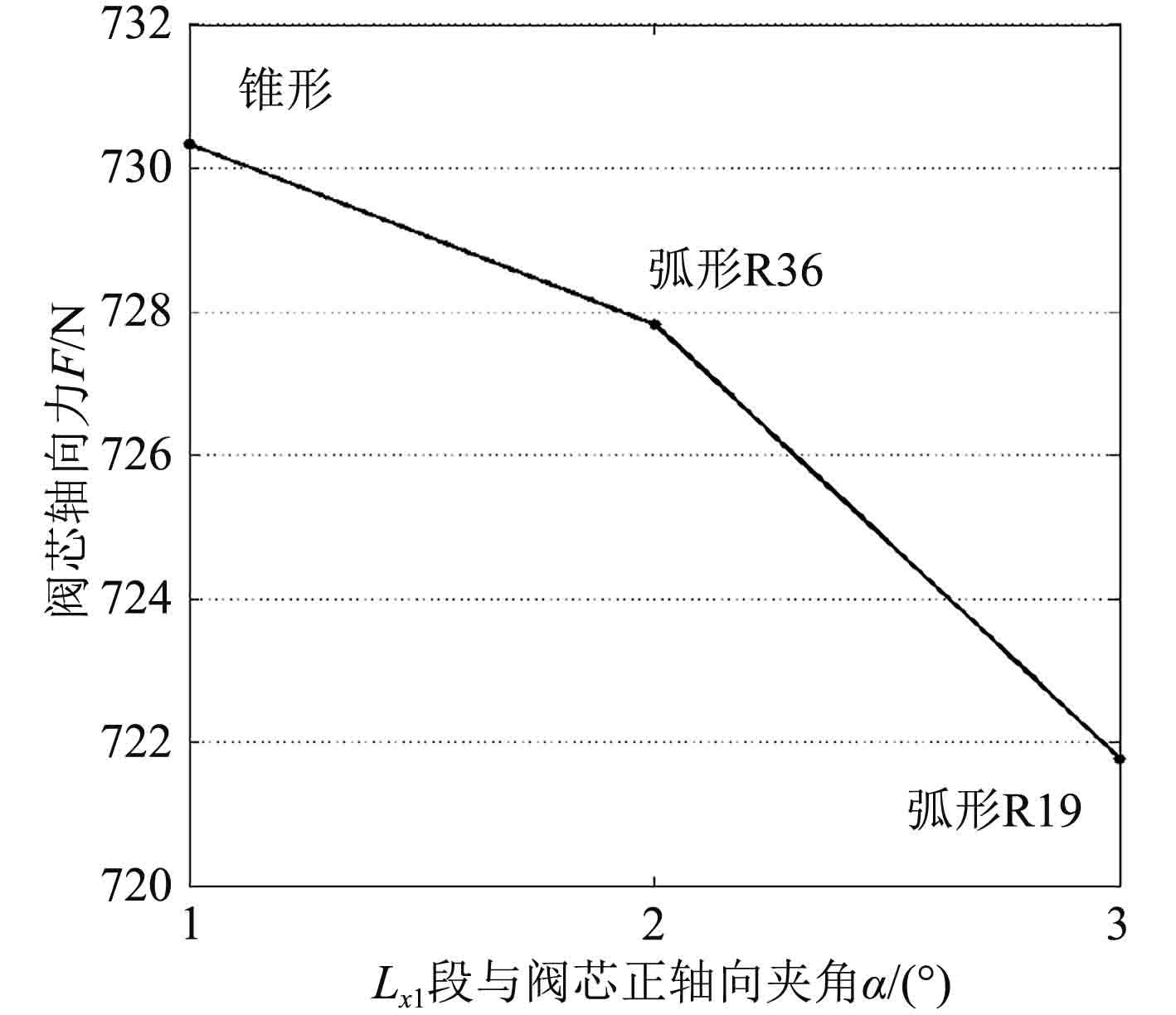图 6 阀芯轴向液压力与Lx2曲线形状关系曲线图 Fig. 6 The relationship curve of valve axial hydraulic force and Lx2 curve shape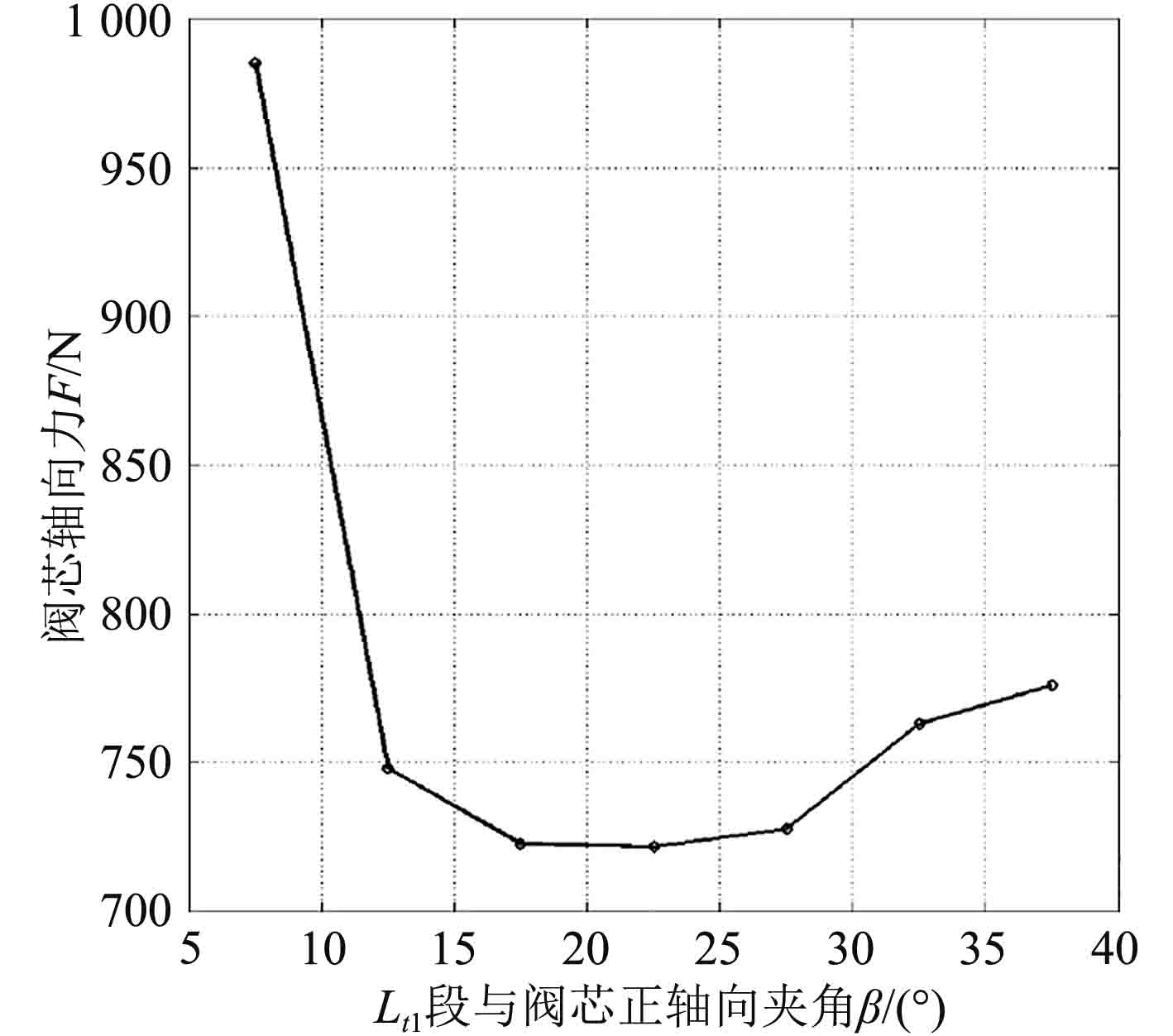图 7 阀芯轴向液压力与夹角β的关系曲线图 Fig. 7 The relationship curve of valve axial hydraulic pressure and angle β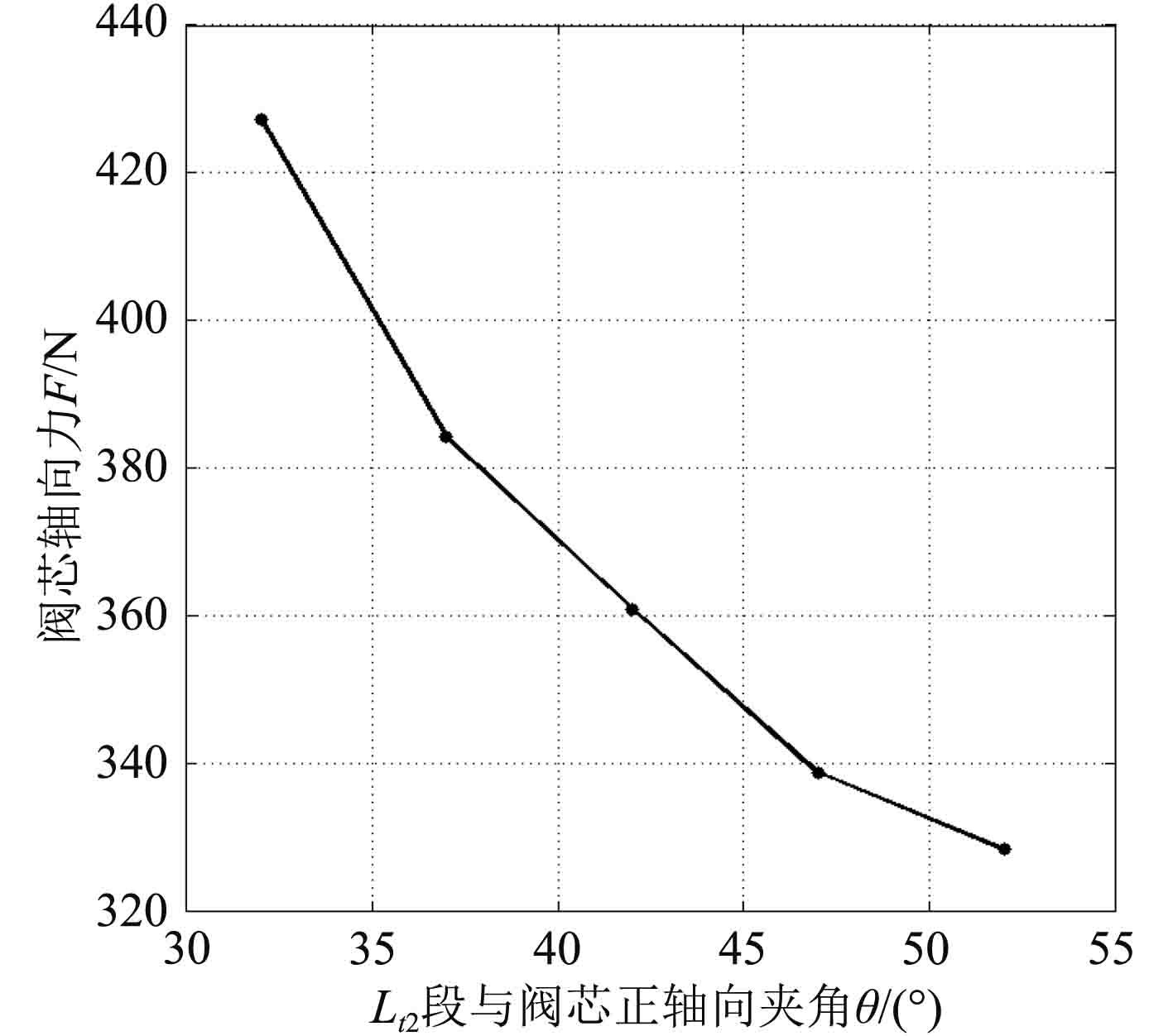图 8 阀芯轴向液压力与夹角Θ的关系曲线图 Fig. 8 The relationship curve of valve axial hydraulic force and angle Θ

1.3 限速切断阀模拟装配及生产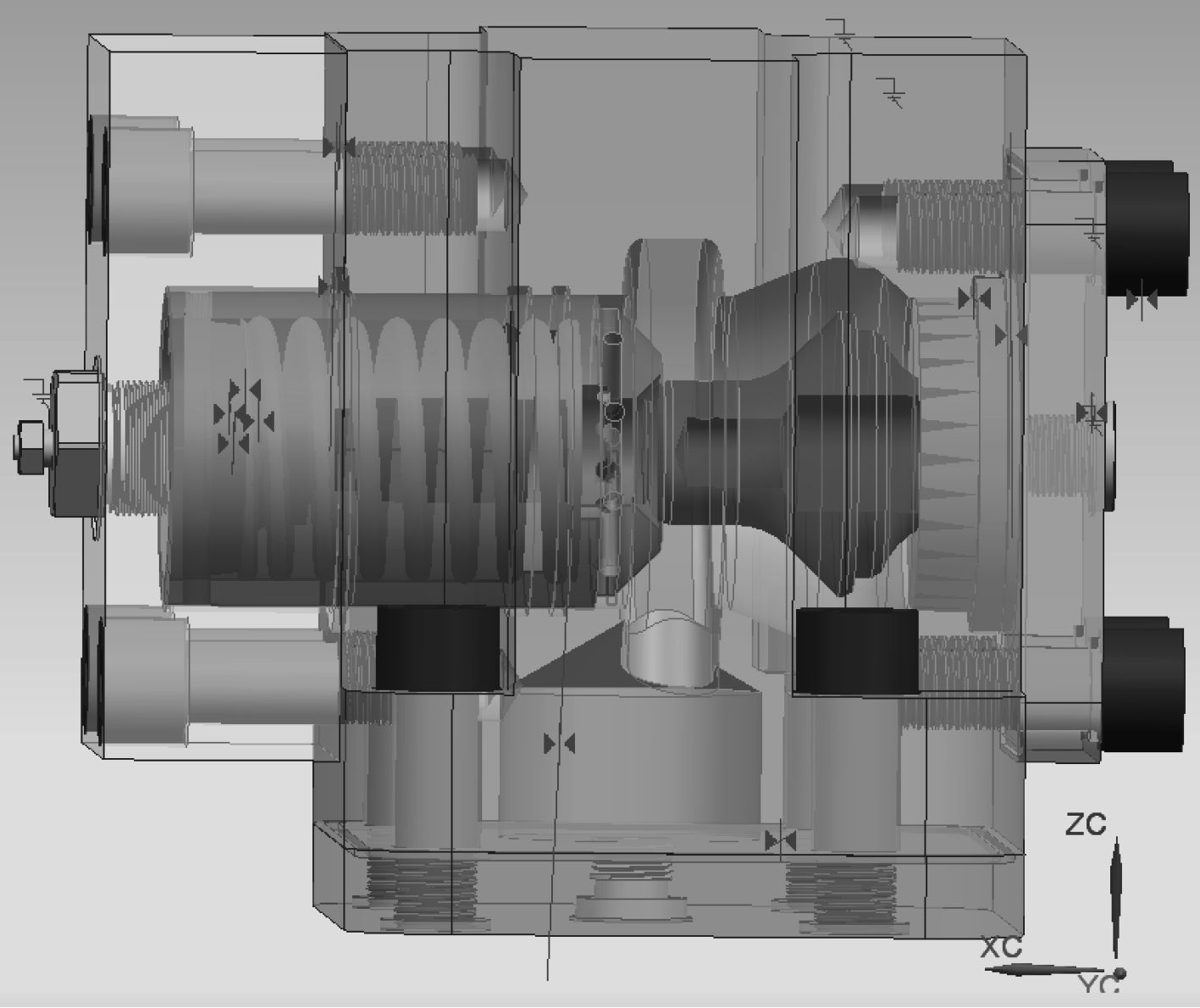图 9 限速切断阀模拟装配图 Fig. 9 Simulation assembly drawing of speed limiting shut-off valve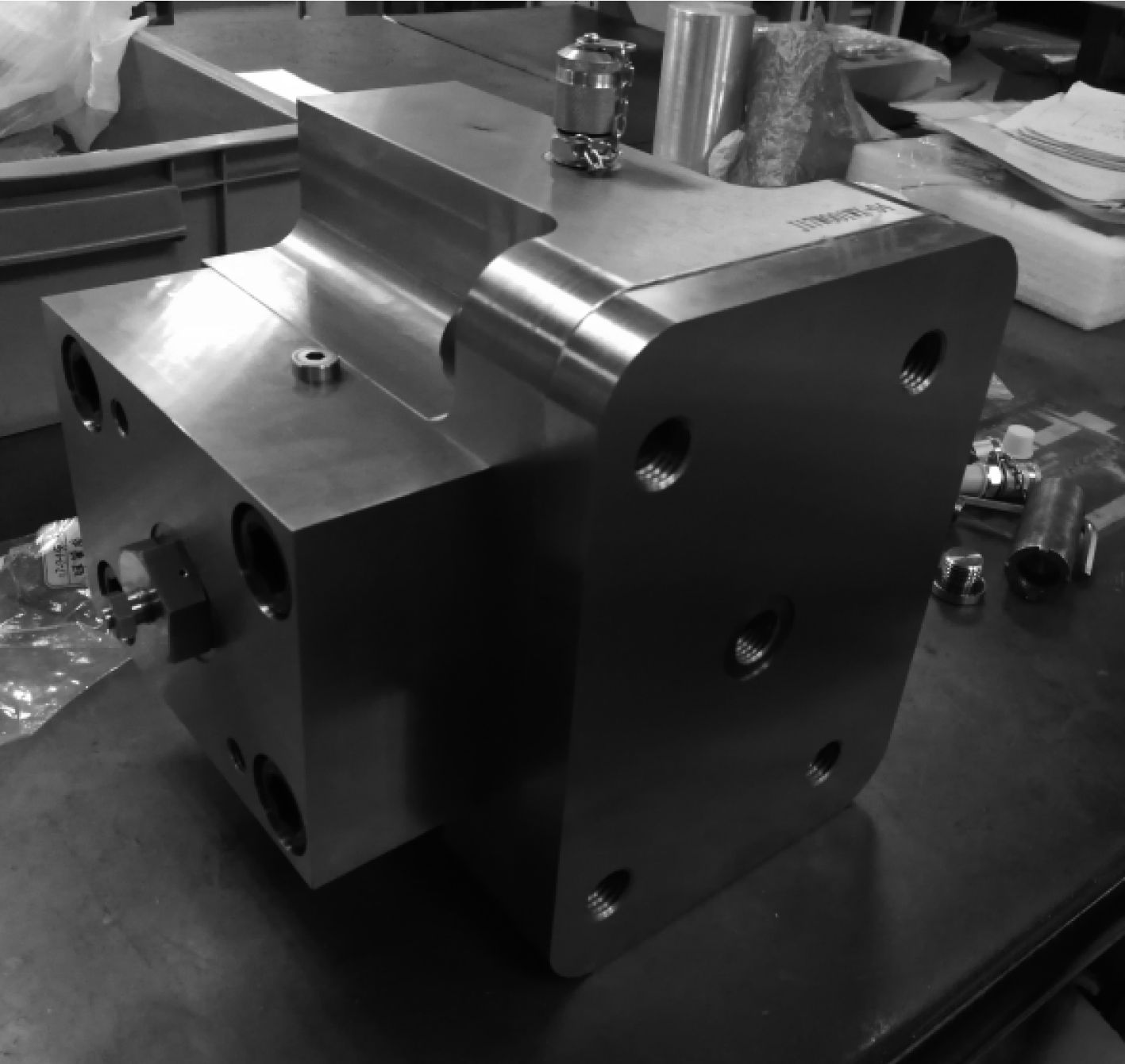图 10 限速切断阀实物装配图 Fig. 10 Actual assembly drawing of speed limiting shut-off valve
2 限速切断阀的试验分析研究

2.1 试验系统的搭建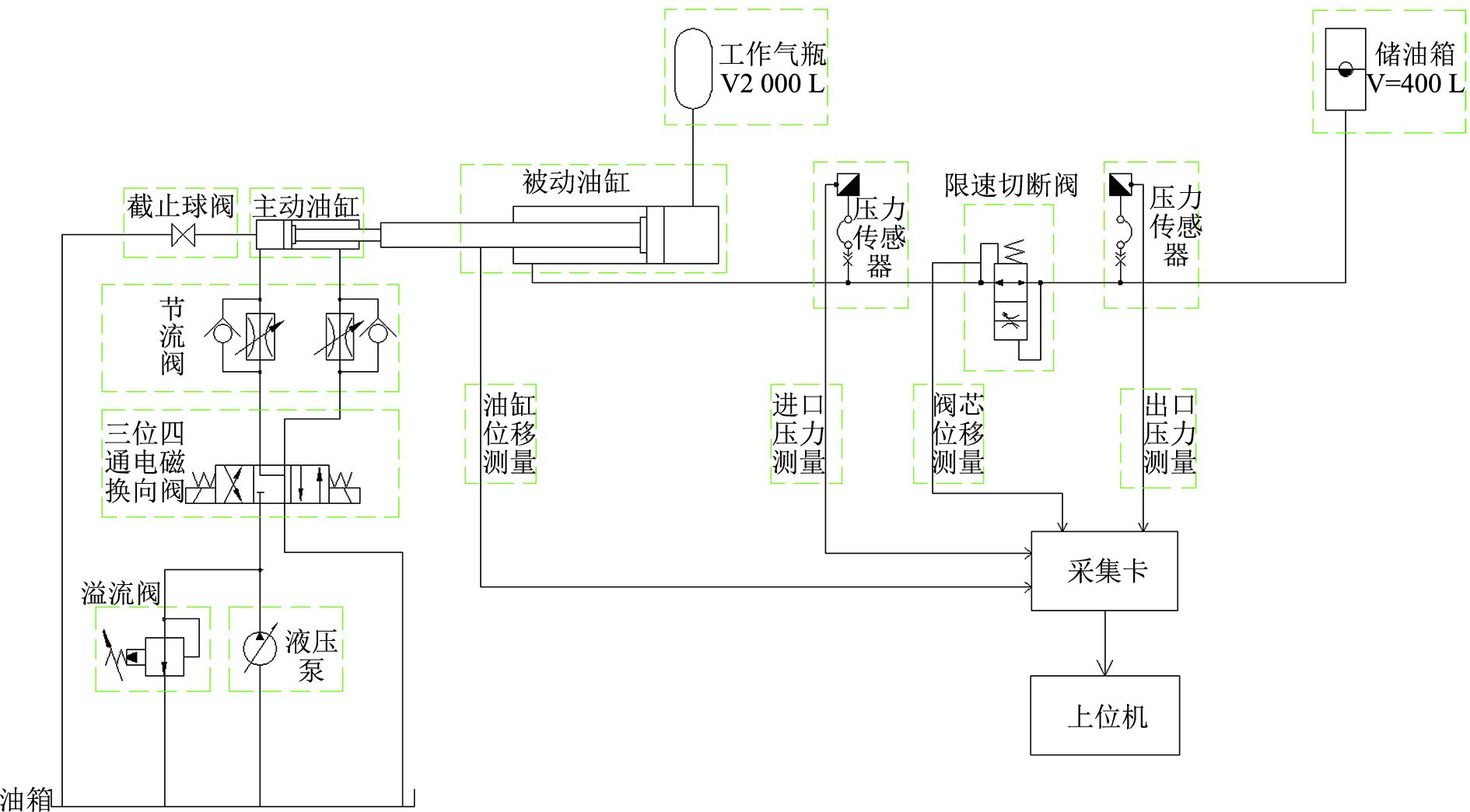图 11 限速切断阀试验系统原理图 Fig. 11 Test system schematic of speed limiting shut-off valve
2.2 不同前阻尼孔直径试验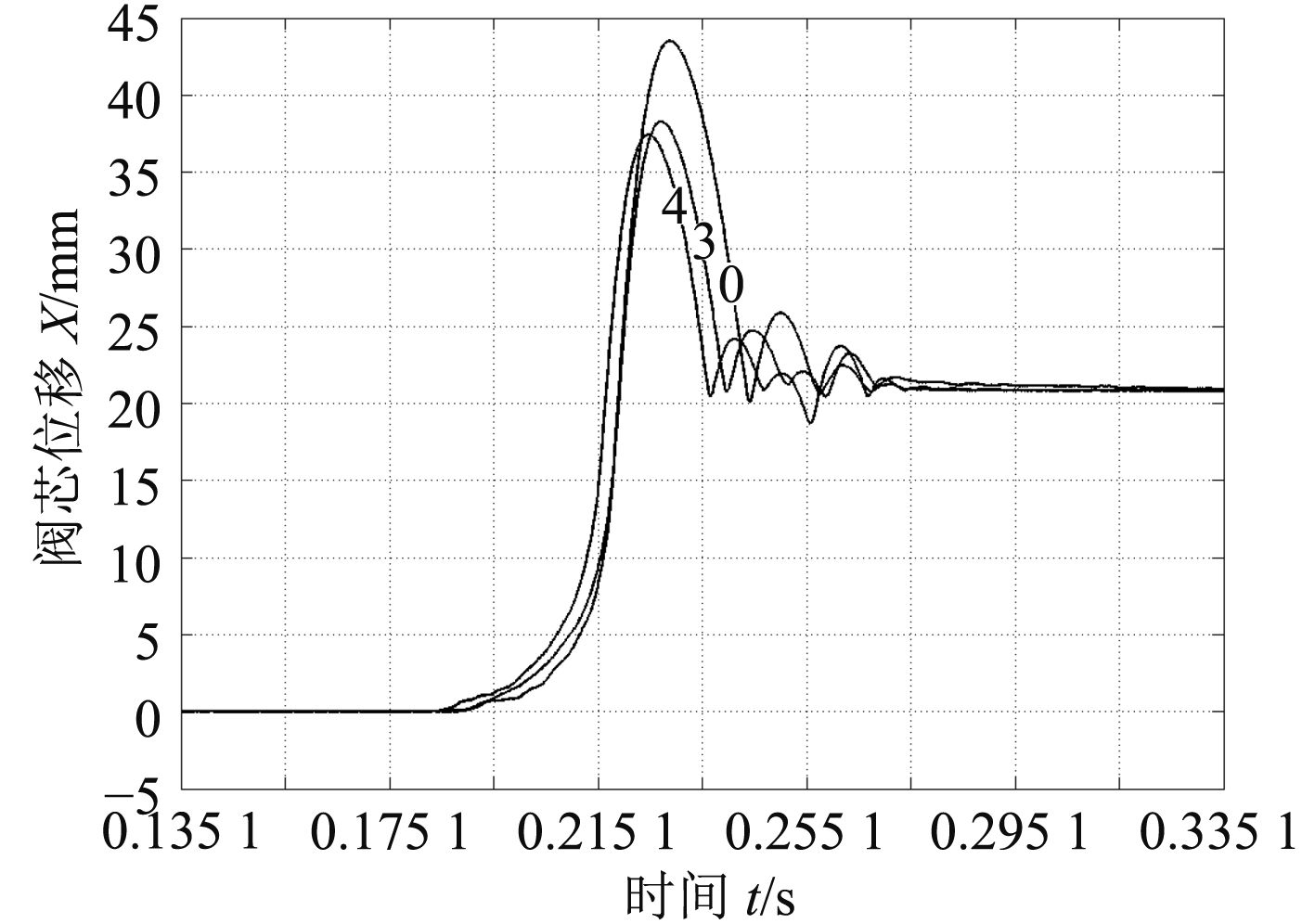图 12 限速切断阀阀芯位移图 Fig. 12 Spool displacement curve of speed limiting shut-off valve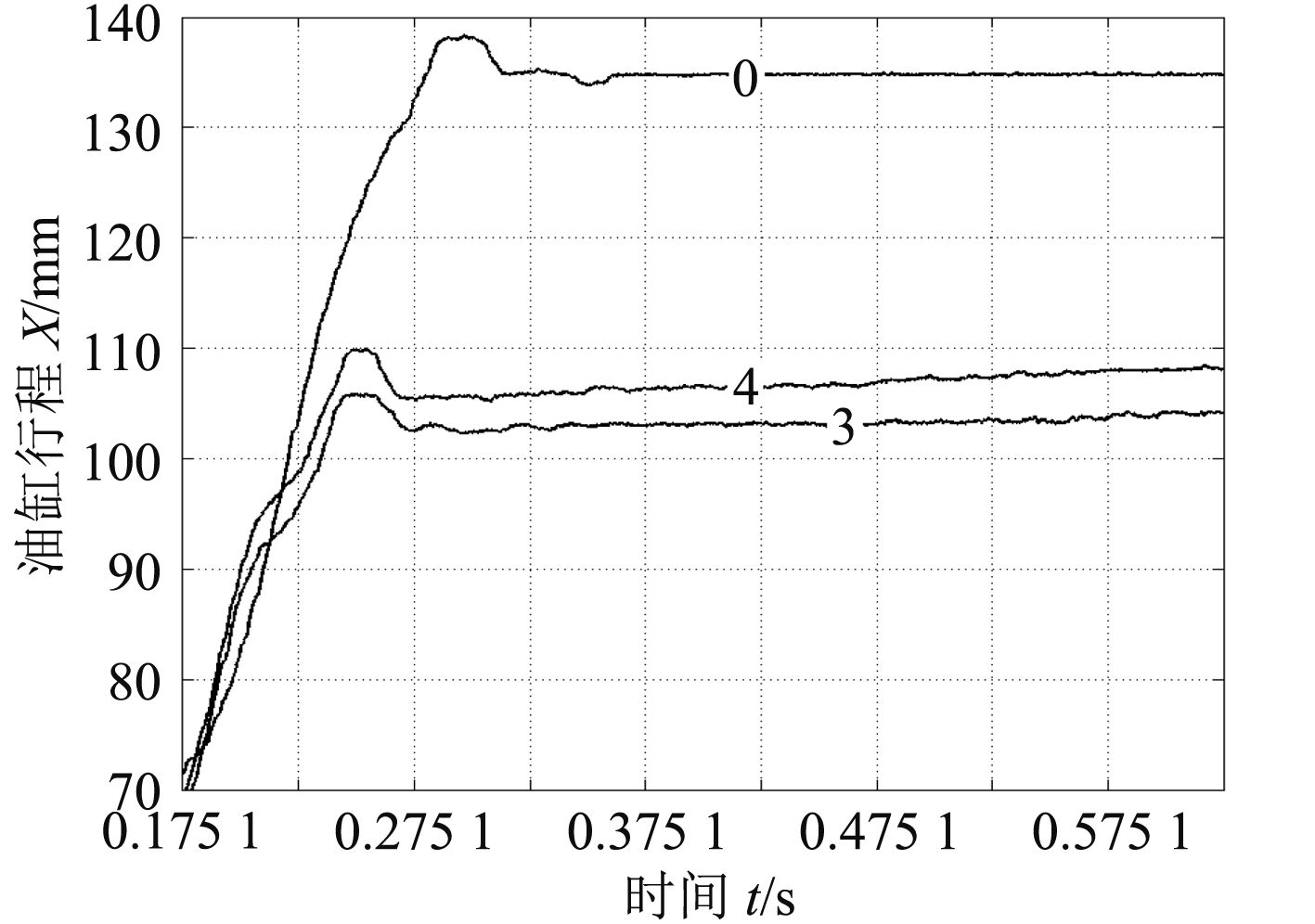图 13 被动油缸活塞杆位移图 Fig. 13 Displacement diagram of passive cylinder piston rod

2.3 不同后阻尼孔直径试验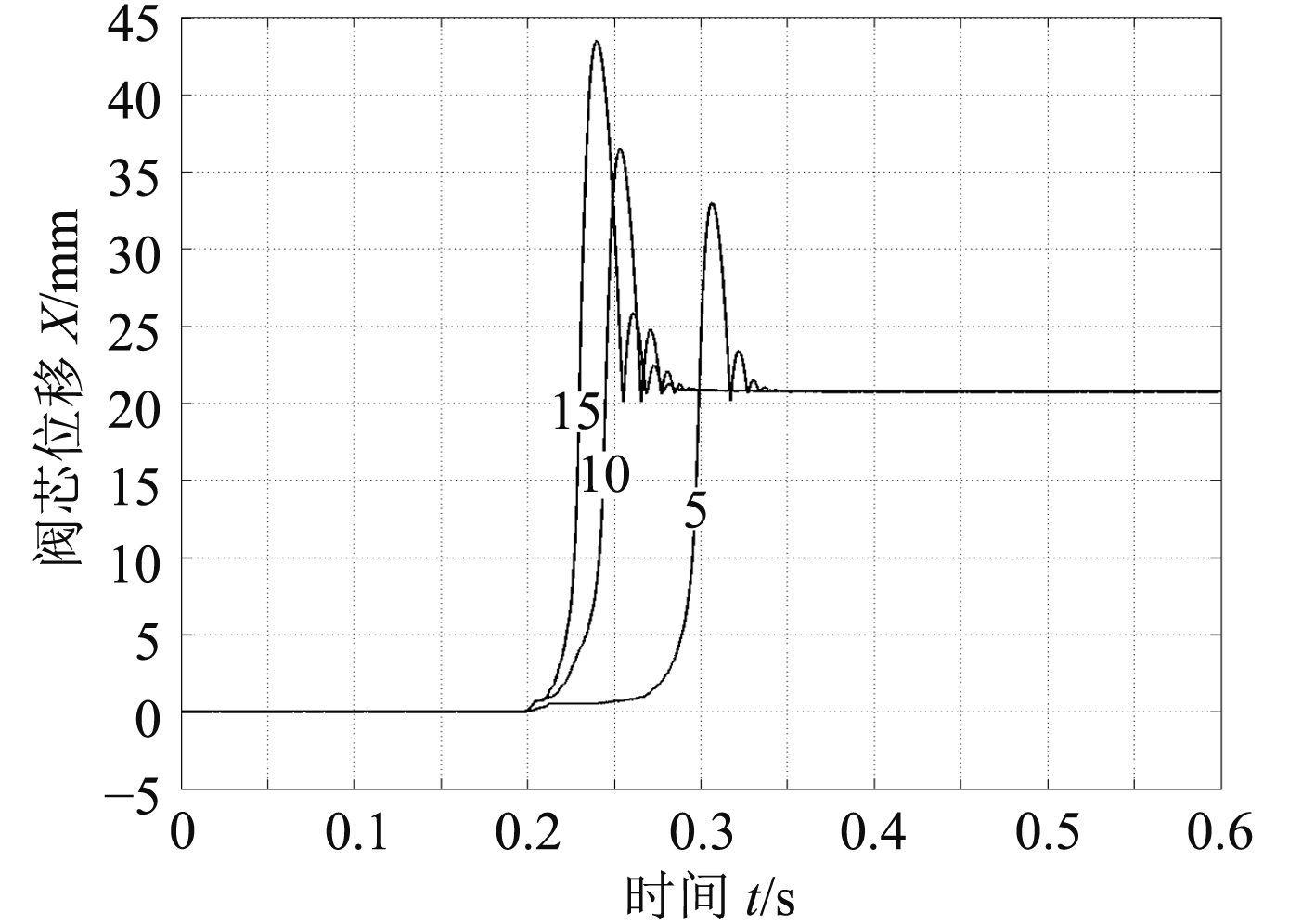图 14 限速切断阀阀芯位移图 Fig. 14 Spool displacement curve of speed limiting shut-off valve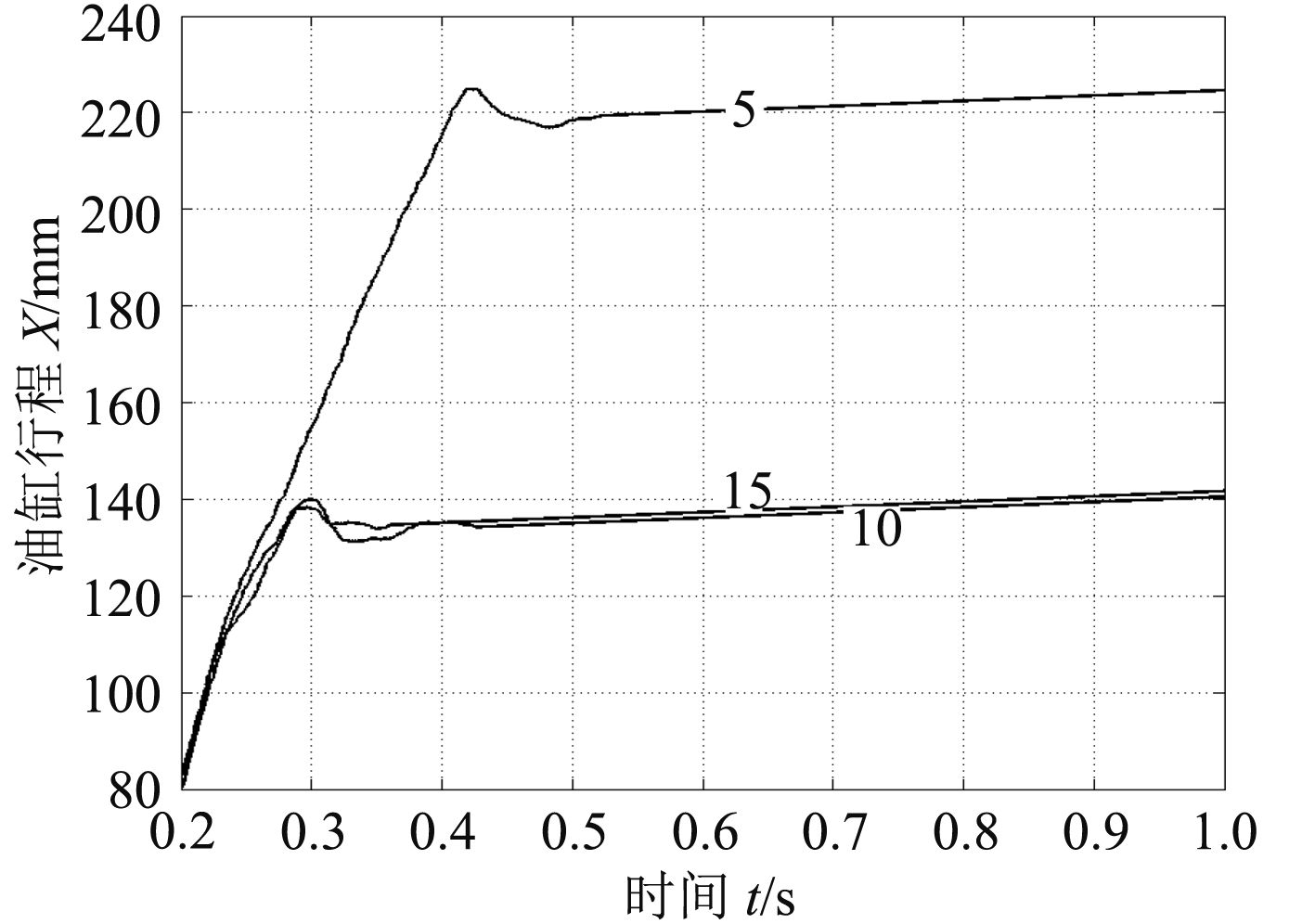图 15 被动油缸活塞杆位移图 Fig. 15 Displacement diagram of passive cylinder piston rod

3 结　语

1）限速切断阀在最佳配置下，切断流量在2 200 L/min左右，切断时间在30 ms左右，基本满足海洋工程装备系统对限速切断阀提出的性能要求。

2）限速切断阀的前阻尼孔直径大小影响限速切断阀本身和气液缸的响应：随着前阻尼孔直径的增大，限速切断阀的关闭速度有所加快，气液缸的运动速度加快，气液缸有杆腔压力峰值减小；同时前阻尼孔直径过大将导致气液缸的速度峰值过大，前阻尼孔直径过小将导致气液缸不能运动到其机械限位处停止。

3）限速切断阀的后阻尼孔直径大小对限速切断阀本身和气液缸的响应有重大影响：当后阻尼孔直径过小时限速切断阀不能正常工作，同时也会失去保护阀控液压缸系统的作用，液压缸将以较大的速度冲顶产生破坏；随着限速切断阀后阻尼孔的增大，限速切断阀的关闭速度加快，但当后阻尼孔直径过大时，将导致系统不稳定，限速切断阀关闭时产生较大波动，实际关闭时间延长；同时后阻尼孔直径过大或过小，都将导致气液缸有杆腔压力峰值过高，不利于系统保护。

  王海波. 水下拖曳升沉补偿液压系统及其控制研究[D]. 杭州: 浙江大学, 2009.  王洁, 官忠范, 范广民. 限速切断阀的设计计算及其典型应用[J]. 沈阳工业大学学报, 1997(5): 34–38. http://www.cnki.com.cn/Article/CJFDTotal-SYGY705.008.htm  徐兵, 刘贺, 杨华勇, 等. 液压电梯限速切断阀的设计[J]. 机床与液压, 2004(10): 17–19.  胡国良, 徐兵, 杨华勇, 等. 新型限速切断阀的设计及实验研究[J]. 浙江大学学报(工学版), 2004, 38(5): 625–630. http://www.cnki.com.cn/Article/CJFDTOTAL-ZDZC200405022.htm  湛大强. 限速切断阀型式试验系统设计及研究[D]. 杭州: 浙江大学, 2009.  宋鸿尧. 液压阀设计与计算[M]. 北京: 机械工业出版社, 1982.